• import pandas as pd import matplotlib.pyplot as plt def getData(): r""" 使用numpy构造满足条件的随机数() :return: """ dataX = np.random.randint(1,100,size=(1,100)) dataY = np.random.randint(1,...

import numpy as np
import pandas as pd
import matplotlib.pyplot as plt

def getData():
r"""
使用numpy构造满足条件的随机数()
:return:
"""

dataX = np.random.randint(1,100,size=(1,100))
dataY = np.random.randint(1,100,size=(1,100))
data = np.concatenate((dataX,dataY), axis = 0)

G1tfa = data > 50
G1tfi = np.logical_and(G1tfa[0,:], G1tfa[1,:])
G1index = np.nonzero(G1tfi)
G1 = data[:, G1index]

G2tfa = data < 50
G2tfi = np.logical_and(G2tfa[0,:], G2tfa[1,:])
G2index = np.nonzero(G2tfi)
G2 = data[:, G2index]

return (G1, G2)

def getDate2():
r"""
使用numpy生成随机数；
使用pandas构造满足条件的随机数；
:return:
"""
df = pd.DataFrame()
df['X'] = np.random.randint(1,100,size=(100))
df['Y'] = np.random.randint(1,100,size=(100))

G1 = df[(df['X']>50) & (df['Y']>50)]
G2 = df[(df['X']<50) & (df['Y']<50)]

return (G1.values.T, G2.values.T)

if __name__ == "__main__":
print("线性回归模型")
G1, G2 = getData()
fig, ax = plt.subplots()
ax.scatter(G1[0,:], G1[1,:])
ax.scatter(G2[0,:], G2[1,:])
fig.show()


展开全文• ids = '10_6610' values = [[1, 2, 3, 4, 5, 6]...# 构造id ids_li = [] for i in range(len(values)): ids_li.append(ids) # 横向分组转为纵向分组 zdict = {} zdict['alpos_id'] = ids_li for i in range(lens):
ids = '10_6610'
values = [[1, 2, 3, 4, 5, 6], [2, 3, 4, 5, 6, 7], [3, 4, 5, 6, 7, 8]]
lens = len(values)

# 构造id
ids_li = []
for i in range(len(values)):
ids_li.append(ids)

# 横向分组转为纵向分组
zdict = {}
zdict['alpos_id'] = ids_li
for i in range(lens):
zdict[i] = values[i]

da_gb = pd.DataFrame(zdict)
da_gb.columns = ['id', 'a', 'b', 'c']

da_gb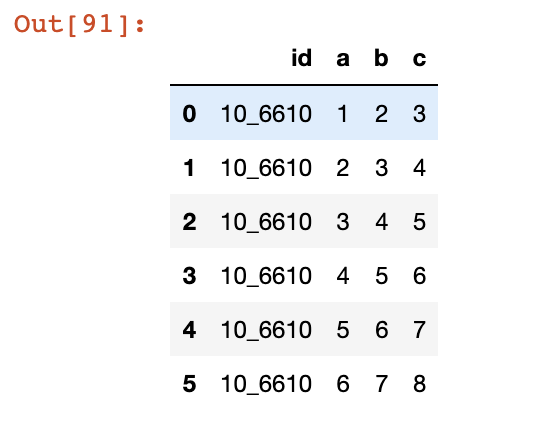展开全文• ## Pandas-DataFrame构造

千次阅读 2017-10-19 17:26:59
一. DataFrame的构造方式 ...1. 通过list或numpy数组构造DF # -*- coding:utf-8 -*- import pandas as pd df = pd.DataFrame([data = ['a', 'b', 'c'], ['d', 'e', 'f'],['g', 'h', 'i']], columns = ['fi...
一. DataFrame的构造方式
1. 通过list或numpy数组构造DF

# -*- coding:utf-8 -*-
import pandas as pd

df = pd.DataFrame([data = ['a', 'b', 'c'], ['d', 'e', 'f'],['g', 'h', 'i']],
columns = ['field1', 'field2', 'field3'])
这是最简单的创建形式，传入的list一般是多维度的，且每一维度元素数目如果不一致，则DataFrame默认字符型缺失值为None，数值型缺失值为NaN（not a number）；当list中传入的数据类型不一样时，遵循如下规则：        ① list中如果有string类型则默认DataFrame该列为string类型        ② list中如果是整数和小数，则小数对应的列是float类型     columns指定的是列名（也就是之前结构中的字段名）。columns参数传入的sequence的长度与多维数组中的列数等长，否则会报错。     其他创建DataFrame的参数还有：         ① index（索引），他在官方文档中的定义为’Index to use for resulting frame. Will default to np.arange(m) ifno indexing‘，即默认是从0到m-1 (行数-1)。也可以自行设置一个序列用于作为索引，该序列中的元素数目必须与行数一致。         ② dtype（类型），在官方文档中的解释为’Data type to force, otherwise infer‘，即用于强制转换数据类型，如果不指定则由Python自行推导。dtype的强制转换能力不是无限的，不可能将string转换为数值类。
2. 通过元组构造DF
形式与list构建DataFrame相近，只是将list换成了tuple类型。
3. 通过字典构造DF

# -*- coding:utf-8 -*-
import pandas as pd

df = pd.DataFrame({'goods':['coke cola', 'eggplant','condom'],
'quantity':[12,3,1],
'price':[20,12,80]})
简单的来说，利用Python的字典构造DataFrame就是讲DataFrame类中的data和columns参数合二为一，键值就是生成的DataFrame的列名。由于Python的字典是无序的，所以在不指定列的顺序的情况下，dict生成的DataFrame的列名顺序是随机的，如果列的顺序很重要，那么久再次对DataFrame中的columns参数进行设置，将字典中原有的键值进行排序。注意，此时上传的columns必须与dict中的键值一致，否则生成失败。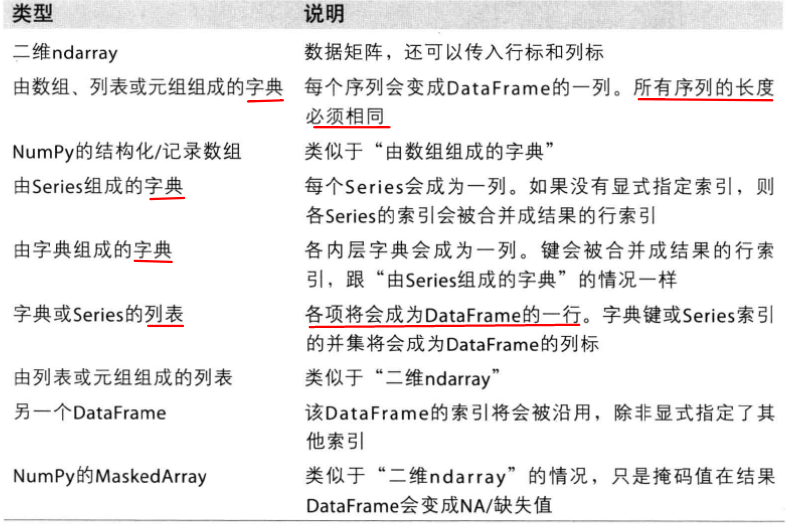二. 生成数据的预览
1. shape属性
#-*- coding:utf-8 -*-
import pandas as pd
import MySQLdb as mdb

conn = mdb.connect(host = 'localhost',port=3306,user= 'root',passwd='*****',db = 'test')
df.shape
shape属性返回的是一个tuple，二维的DataFrame显示的是(x,y)，其中x是行数，y是列数。Pandas的Dataframe的shape属性输出的不是索引，而是实实在在的几行几列，所以要格外注意。
2. columns属性
#-*- coding:utf-8 -*-
import pandas as pd
#-*- coding:utf-8 -*-
import pandas as pd
import MySQLdb as mdb

conn = mdb.connect(host = 'localhost',port=3306,user= 'root',passwd='*****',db = 'test')
df.columns

返回的形式是 Index(['column1','column2',...'colmun N'])这样的形式，显示的是各列的名字。
3.describe属性
#-*- coding:utf-8 -*-
import pandas as pd
import MySQLdb as mdb

conn = mdb.connect(host = 'localhost',port=3306,user= 'root',passwd='*****',db = 'test')
df.describe
describe()方法用于描述DataFrame，其中有个 include参数，写入的是字符串或者一个列表，里面的元素是要查看的数据类型。
4. name属性
dataframe的索引和列都是可以给定名字的，使用的是 index和 columns的 name属性，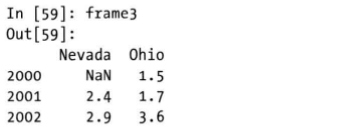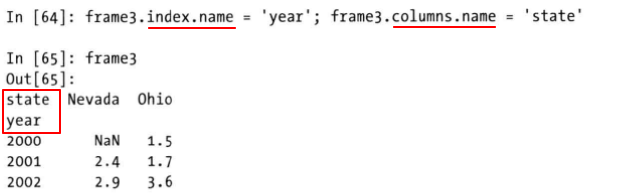5. 转换为Numpy数组
与Series一样，DataFrame的values属性能够将DataFrame转换为二维numpy数组的形式，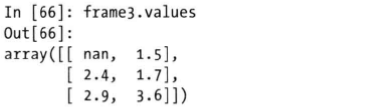这个例子中，DataFrame的所有数据类型都是相同的，实际上，即使DataFrame数据类型不同，values属性也能将DataFrame转换为兼容所有数据类型的数组。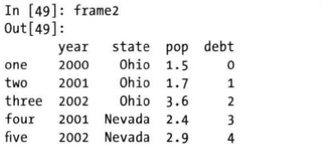如在frame2中，各列的变量类型是不同的，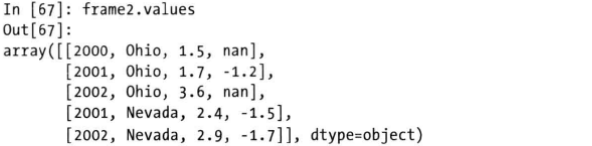三. DataFrame运算

1. DataFrame之间的运算
运算时，行索引和列索引都要匹配上才能进行运算，否则得到的是缺失值，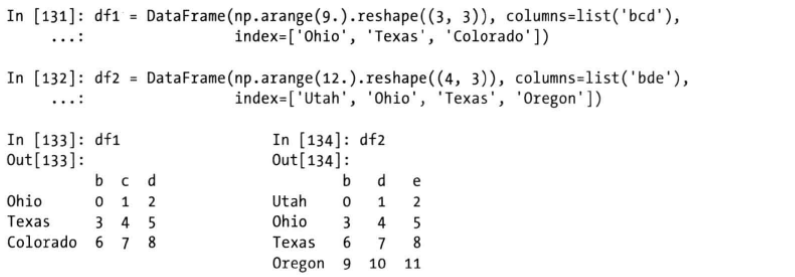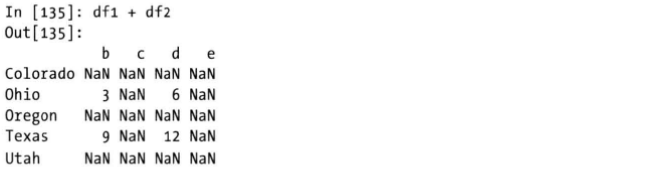但是在一些时候，运算产生的缺失值是不希望继续传播的，此时可以使用Pandas提供的一些方法，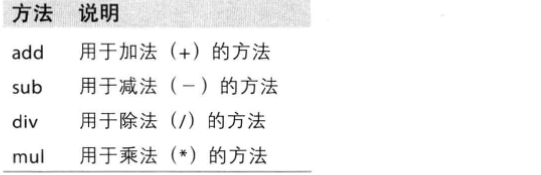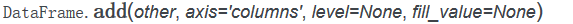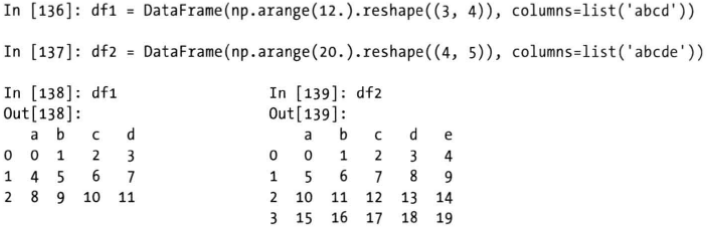对于上面两个DataFrame，使用普通的运算符进行运算，没有行列匹配的元素会得到NaN的值，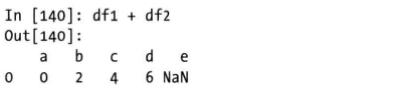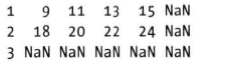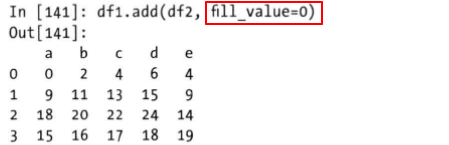2. Series与DataFrame之间的运算

pandas基于numpy，所以dataframe和series之间运算时，会有和numpy数组一样的广播效应，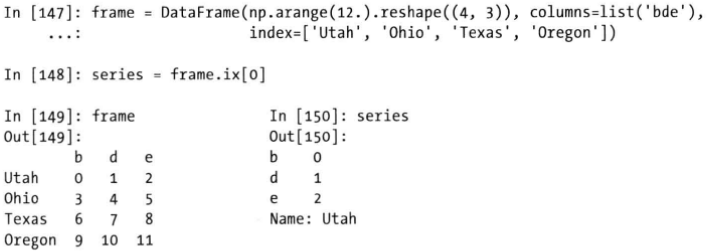Series的索引会默认找到Dataframe中匹配的列，然后竖直方向传播。如果Series的索引在Dataframe的列索引中不存在，则Dataframe和Series会被重新索引，新的列索引是二者索引的并集，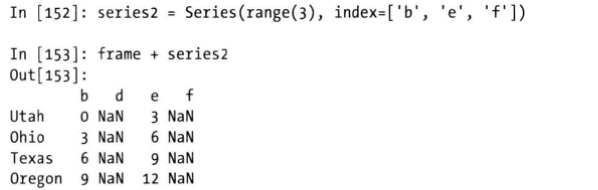运算后，匹配不上的结果会最终形成缺失值。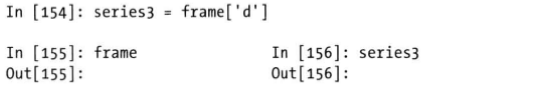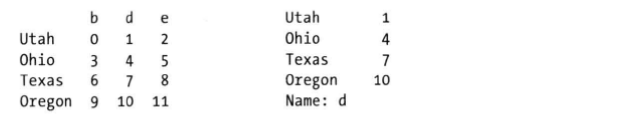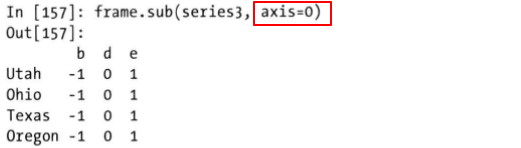展开全文DataFrame 数据 Python
• pandas学习之df.loc and df.iloc 在数据处理中，经常需要从数据集中提取出部分数据，这种情况下，可以选择df.loc or df.iloc这两种方法。这种方法和df[[列名列表]]类似，但效率上会高于df和列名列表的组合方式。 .....
pandas学习之df.loc
在数据处理中，经常需要从数据集中提取出部分数据，这种情况下，可以选择df.loc or df.iloc这两种方法。这种方法和df[[列名列表]]类似，但效率上会高于df和列名列表的组合方式。 可以做一个简单的比较：
df.shape#df数据集的概况
(51290, 24)
# %timeit 用于测试一段程序运行效率
%timeit df[['Order_Date','Customer_ID','Market']]#df加列名的提取方式
2.85 ms ± 109 µs per loop (mean ± std. dev. of 7 runs, 100 loops each)

%timeit df.loc[:,['Order_Date','Customer_ID','Market']]#df.loc的方式
2.6 ms ± 115 µs per loop (mean ± std. dev. of 7 runs, 100 loops each)
#数据集越大，差距越明显

df.loc篇：
官方文档：
Access a group of rows and columns by label(s) or a boolean array. .loc[] is primarily label based, but may also be used with a boolean array. Allowed inputs are:
A single label, e.g. 5 or ‘a’, (note that 5 is interpreted as a label of the index, and never as an integer position along the index).A list or array of labels, e.g. [‘a’, ‘b’, ‘c’].A slice object with labels, e.g. ‘a’:‘f’. Warning Note that contrary to usual python slices, both the start and the stop are includedA boolean array of the same length as the axis being sliced, e.g. [True, False, True].A callable function with one argument (the calling Series or DataFrame) and that returns valid output for indexing (one of the above)
解释：
需要传入行列或者布尔值的数组：.loc[]不仅允许传入基于标签的数组，还可以是布尔值数组允许的输入类型为：
单个标签，比如‘5’，‘a’。需要注意的是5是行索引上的整数型标签，而不是索引上的第5行，比如有3把椅子，坐着3个人，名字分别是一二三，第3把椅子坐着的是三。3代表这个人处于位置，无论谁坐在这，这里都是第3把椅子；三是这个人名字，无论他在什么位置，他的名字都是三。而.loc[]就是基于标签（名字）来提取的一个标签的列表[‘a’,‘s’]一个标签的切片，如‘a’：‘f’ 注意： .loc[]的切片和python中的不同，这里的切片两端都包括，即左闭右闭区间，而python中是左闭右开区间一个布尔值数组，需要和被切片的轴长度一致一个函数
实验：
import pandas as pd
import numpy as np
df = pd.DataFrame({'name':['张三','李四','王二','麻子','杜甫'],'mark':[120,111,135,150,151],'gender':['male','female','female','male',np.nan]})
df
#为方便演示，构造一个简单的dataframe
name	mark	gender
0	张三	120		male
1	李四	111		female
2	王二	135		female
3	麻子	150		male
4	杜甫	151		NaN

#单个标签
df.loc[:,'name']#前面的冒号相当于一个切片，即选取所有行，如果直接df.loc['name']会报错
0    张三        #所以可以理解为df.loc[对行的操作，对列的操作]，对列可以省略，行不可以
1    李四
2    王二
3    麻子
4    杜甫
Name: name, dtype: object

df.loc
name        张三
mark       120
gender    male
Name: 0, dtype: object

#标签列表，注意要使用 [[]] 的形式
df.loc[[0,2],'name']
0    张三
2    王二
Name: name, dtype: object

#切片操作，两端都可以取到
df.loc[0:2,'name'] #注意，这里的0：2是标签，不是位置序列！！！
0    张三
1    李四
2    王二
Name: name, dtype: object

df.loc[1,'name':'gender']# 这样可能更直观，这是个切片是标签
name          李四
mark         111
gender    female
Name: 1, dtype: object

#可以传入一个布尔值列表
df.loc[:,[False,True,False]]#注意列表长度必须和对应得轴长度一致，否则报错
mark
0	120
1	111
2	135
3	150
4	151

#可以输入条件，筛选出需要的数据
df.loc[df['mark']>=135]
name	mark	gender
2	王二	135		female
3	麻子	150		male
4	杜甫	151		NaN

#也可以是函数
df.loc[lambda df: df['mark'] >= 150]
name	mark	gender
3	麻子	150		male
4	杜甫	151		NaN

#也可以直接对结果赋值
df.loc[4,'gender'] = 'female'
df
name	mark	gender
0	张三	120		male
1	李四	111		female
2	王二	135		female
3	麻子	150		male
4	杜甫	151		female

6.18学习打卡
加油！！！学习虽然不一定会变强，但一定会便变秃
展开全文python 大数据
• 目录 零、Pandas 基本介绍 一、Series - 一维数据结构 二、DataFrame - 二维数据结构 2-1 二维数据构造方式 2-1-2 从dict 构造 ...2-1-3 从 python list 构造 ...2-1-4 从 numpy ndarray ...3-0 DF构造 3-1 DF.T.to_...
• 一、Series 1、 np.random.randn(m,n)/np.random.rand(m,n)生成的是一...df = pd.Series(np.random.randn(5), index=['a', 'b', 'c', 'd', 'e']) a -2.254714 b -0.093877 c 0.003537 d -0.753373 e 1.270707 dty.python 数据挖掘 人工智能
• ## Pandas

2017-06-22 14:38:18Python
• 1.官网学习 ...为了后面演示方便，我现在会提前构造出一些数据，这些数据随手构造的，基本和平时用到的数据格式是一样的，如果觉得我造的数据比较丑，大家可以按照自己的喜好重新构造。下面介绍一下数据...Python 数据合并
• ## pandas

2021-08-06 19:51:21
1.pandas是什么 官网：https://pandas.pydata.org/ 源码：https://github.com/pandas-dev/pandas 开发文档：https://pandas.pydata.org/pandas-docs/stable/reference/io.html Pandas 是 Python 语言的一个扩展程序...
• # 构造id ids_li = [] for i in range(len(values)): ids_li.append(ids) # 横向分组转为纵向分组 zdict = {} zlis = [] zdict['alpos_id'] = ids_li for i in range(lens): ...python
• 注：既然很多数据可以从numpy的array转换过来，pandas的数据构造就可以参照numpy的数据构造， 参考博文：https://blog.csdn.net/u010916338/article/details/105325982 1.3通过direct字典转换 ...
• 如果我尝试以下方法： df = pandas.DataFrame(users_summary) “级别1”（用户ID）中的项目被视为列，这与我想要实现的相反（将用户ID作为索引）。 我知道我可以在迭代字典条目之后构造这个系列，但是如果有更直接...
• 以上图片截取自利用python进行数据...构造DataFrame的数据格式 import pandas as pd import numpy as np 二维ndarray arr2d = np.random.randint(0,9,size=(5,4)) arr2d array([[6, 3, 0, 4], [2, 5, 5, 0], ......